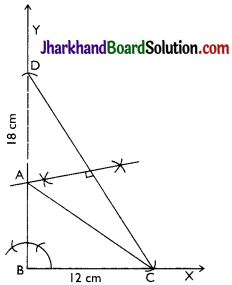# JAC Class 9 Maths Solutions Chapter 11 Constructions Ex 11.2

Jharkhand Board JAC Class 9 Maths Solutions Chapter 11 Constructions Ex 11.2 Textbook Exercise Questions and Answers.

## JAC Board Class 9th Maths Solutions Chapter 11 Constructions Ex 11.2

Page-195

Question 1.
Construct a triangle ABC in which BC = 7 cm, ∠B = 75° and AB + AC = 13 cm.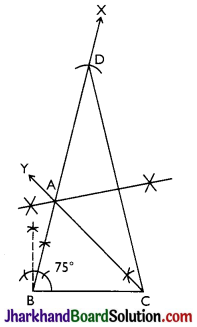Steps of Construction:
Step 1: A line segment BC of 7 cm is drawn.
Step 2: At point B, an angle ∠XBC is constructed such that it is equal to 75°.
Step 3: A line segment BD =13 cm is cut on BX (which is equal to AB + AC).
Step 4: DC is joined.
Step 5: Draw perpendicular bisector of CD which meets BD at A.
Step 6: Join AC.
Thus, ∆ABC is the required triangle.

Question 2.
Construct a triangle ABC in which BC = 8 cm, ∠B = 45° and AB – AC = 3.5 cm.
Steps of Construction:
Step 1: A line segment BC = 8 cm is drawn and at point B, make an angle of 45° i.e. ∠XBC.
Step 2: Cut the line segment BD = 3.5 cm (equal to AB – AC) on ray BX.
Step 3: Join DC and draw the
perpendicular bisector PQ of CD.
Step 4: Let it intersect BX at point A. Join AC.
Thus, ∆ABC is the required triangle.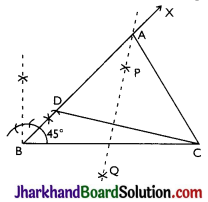Question 3.
Construct a triangle PQR in which QR = 6 cm, ∠Q = 60° and PR – PQ = 2 cm.
Steps of Construction:
Step 1: A line segment QR = 6 cm is drawn.
Step 2: A ray QY is constructed making an angle of 60° with QR and YQ is produced backwards to form a line YY’.
Step 3: Cut off a line segment QS = 2 cm from QY’. RS is joined.
Step 4: Draw perpendicular bisector of RS intersecting QY at a point P. PR is joined. Thus, ∆PQR is the required triangle.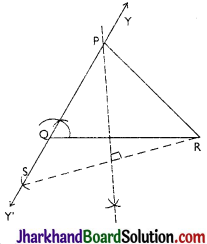Question 4.
Construct a triangle XYZ in which ∠Y = 30°, ∠Z = 90° and XY + YZ + ZX = 11 cm.
Steps of Construction:
Step 1: A line segment PQ = il cm is drawn. (XY + YZ + ZX =11 cm)
Step 2: An angle, ZRPQ = 30° is constructed at point P and an angle ZSQP = 90° at point Q.
Step 3: ZRPQ and ZSQP are bisected. The bisectors of these angles intersect each other at point X.
Step 4: Perpendicular bisectors TU of PX and WV of QX are constructed.
Step 5: Let TU intersect PQ at Y and WV intersect PQ at Z. XY and XZ are joined.
Thus, ∆XYZ is the required triangle.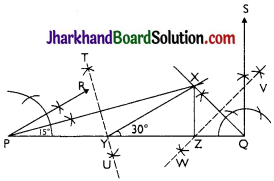Question 5.
Construct a right triangle whose base is 12 cm and sum of its hypotenuse and other side is 18 cm.